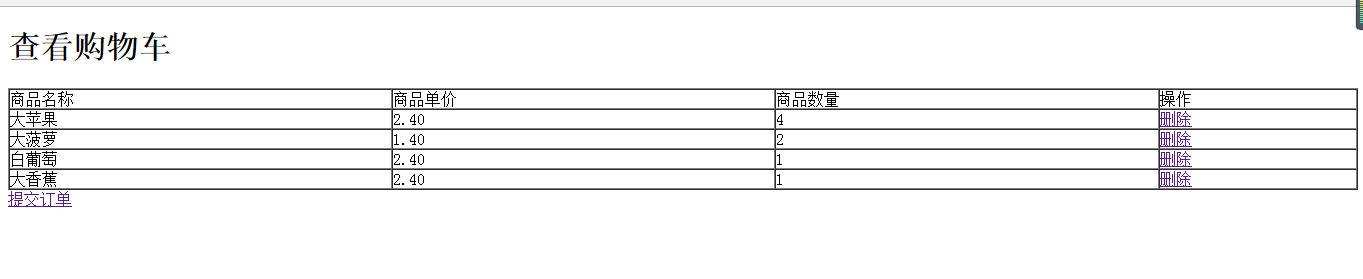# php实现商城购物车的思路和源码分析_php实例\$.post basePath+\"/shoppingcar/selectAll.do\", {}, function(result){ if(result.success){ var obj=result.data;(obj).each(function(index){ var url=\"upload/image/\"+this.car_id+\"/\"+this.<body><h1>登入页面</h1><form action="dengrucl.php" method="post"> <div>帐号：<input type="text" name="zhang"/></div> <div>密码：<input type="text" name="mi"/></div> <input type="submit" value="登入"/></form></body><?phpsession_start();include ("db.class.php");\$db = new db();\$zhang = \$_POST["zhang"];\$mi = \$_POST["mi"];\$sql = "select mi from yonghu WHERE zhang = '{\$zhang}'";\$arr = \$db->Query(\$sql);if(!empty(\$zhang)&&!empty(\$mi)&&\$mi = \$arr){ \$_SESSION["zhang"] = \$zhang; header("location:zym.php");}else{ echo "登入失败";}?>

<body><h1>长腿璇购物商城</h1><table border="1" cellpadding="0" cellspacing="0" width="100%" > <tr> <td>代号</td> <td>水果名称</td> <td>水果价格</td> <td>水果产地</td> <td>水果库存</td> <td>操作</td> </tr> <?php session_start();\$_SESSION["zhang"] = "xiaoming";//不做登入的情况下，直接存sessiion include ("db.class.php"); \$db = new db(); \$sql = "select * from sgbiao"; \$arr = \$db->Query(\$sql); foreach (\$arr as \$v) { echo " <tr> <td>{\$v}</td> <td>{\$v}</td> <td>{\$v}</td> <td>{\$v}</td> <td>{\$v}</td> <td> <a href='zymcl.php?ids={\$v}'>加入购物车</a> </td> </tr>"; } ?> <?php //这里显示的是 购物车有多少产品，和产品的总价格 \$ann=array(); if(!empty(\$_SESSION["gwc"])) { \$ann=\$_SESSION["gwc"]; } \$zhonglei = count(\$ann); \$aa=0; foreach(\$ann as \$k) { \$k;//水果代号 \$k;//水果数量 \$sql1="select jiage from sgbiao where ids='{\$k}'"; \$danjia=\$db->Query(\$sql1); foreach(\$danjia as \$n) { \$aa=\$aa + \$n*\$k; } } echo"数量：{\$zhonglei}<br/>价格:<mark>{\$aa}元"; ?></table><a href="tijiao.php" rel="external nofollow" rel="external nofollow" >查看账户</a><a href="ckgwc.php" rel="external nofollow" >查看购物车</a></body><?phpsession_start();//\$ids = \$_GET["ids"];if(empty(\$_SESSION["gwc"])){ //如果点击的购物车是空的（第一次添加） //如果购物车里是空的，造二维数组， \$arr = array( array(\$ids,1) //一维数组，取ids，第一次点击增加一个 ); \$_SESSION["gwc"]=\$arr; //扔到session里面}else //这里不是第一次点击{ //先判断购物车里是否已经有了该商品，用\$ids \$arr = \$_SESSION["gwc"]; //把购物车的状态取出来 \$chuxian = false;//定义一个变量；用来表示是否出现，默认是未出现 foreach (\$arr as \$v) { //便利他 //如果这里面有这件商品 if (\$v == \$ids) //如果取过来的\$v（商品的代号）等于\$ids那么就证明购物车中已经有了这一件商品 { \$chuxian = true; //如果出现，直接把chuxian改成true } } if(\$chuxian) { //购物车中有此商品 for(\$i=0;\$i<count(\$arr);\$i++) { if(\$arr[\$i] == \$ids) { //把点到的商品编号加1 \$arr[\$i] += 1; } } \$_SESSION["gwc"] = \$arr; } else { //这里就只剩下：购物车里有东西，但是并没有这件商品 \$asg = array(\$ids,1); //设一个小数组 \$arr[] = \$asg; \$_SESSION["gwc"]=\$arr; }}header("location:zym.php")?>

<body><h1>查看购物车</h1><table width="100%" border="1"cellspacing="0" cellpadding="0"> <tr> <td>商品名称</td> <td>商品单价</td> <td>商品数量</td> <td>操作</td> </tr> <?php session_start(); if(!empty(\$_SESSION["gwc"])) { \$arr = array(); \$arr = \$_SESSION["gwc"]; //造数组 } include ('db.class.php'); \$db = new db(); foreach (\$arr as \$v) { global \$db; \$sql = "select * from sgbiao WHERE ids = '{\$v}'"; \$att = \$db->query(\$sql); foreach (\$att as \$a) { echo "<tr> <td>{\$a}</td> <td>{\$a}</td> <td>{\$v}</td> <td><a href='shanchu.php?ids={\$a}'>删除</a> </td> </tr> ";// 蔬果的名称// 单价// 取int数量// 这个地方也可以加索引shanchu.php?sy={\$v} } } ?></table><a href="tijiao.php" rel="external nofollow" rel="external nofollow" >提交订单</a></body><?phpsession_start();\$ids = \$_GET["ids"];\$arr = \$_SESSION["gwc"];//var_dump(\$arr);//取索引2（数量）foreach (\$arr as \$key=>\$v){ if(\$v==\$ids) { if(\$v>1){ //要删除的数据 \$arr[\$key]-=1; } else{ //数量为1的情况下，移除该数组 unset(\$arr[\$key]); } }}\$_SESSION["gwc"] = \$arr;//记得扔到session里面header("location:ckgwc.php");//删除完跳转回去

<?phpsession_start();include ("db.class.php");\$db = new db();//判断用余额是否满足\$zhang = \$_SESSION["zhang"];//获取到用户名\$sye = "select zhanghu from yonghu WHERE zhang = '{\$zhang}'";\$ye = \$db->query(\$sye);\$ye;//这是余额\$ann=array();if(!empty(\$_SESSION["gwc"])){ \$ann=\$_SESSION["gwc"];}\$zhonglei = count(\$ann);\$aa=0;//总价格foreach(\$ann as \$k){ \$k;//水果代号 \$k;//水果数量 \$sql1="select jiage from sgbiao where ids='{\$k}'"; \$danjia=\$db->Query(\$sql1); foreach(\$danjia as \$n) { \$aa=\$aa + \$n*\$k; }}//判断余额是否满足if(\$ye>=\$aa){ //钱够,判断库存 foreach(\$ann as \$v) { \$skc = "select sgname,kucun from sgbiao WHERE ids='{\$v}'"; //水果代号\$v \$akc = \$db->query(\$skc); \$akc;//库存 //比较是否满足库存 if(\$akc<\$v) { echo "{\$akc}库存不足"; //退出 exit; } }//提交订单：//i. 从用户账户中扣除本次购买的总价格//ii. 从商品库存中扣除本次每种商品的购买数量//iii. 向订单表和订单内容表中加入本次购买的商品信息 //扣除账户余额\$skcye = "update yonghu set zhanghu = zhanghu-{\$aa} WHERE zhang = '{\$zhang}'"; \$db->query(\$skcye,0); //扣除库存 foreach(\$ann as \$v) { \$skckc = "update sgbiao set kucun = kucun-{\$v} WHERE ids='{\$v}'"; //水果代号\$v \$db->query(\$skckc,0); } //添加订单信息 //取当前时间 \$time = time(); //自动生成订单号 \$ddh = date("YmdHis"); \$sdd = "insert into dingdan VALUES ('{\$ddh}','\$zhang','\$time')"; \$db->query(\$sdd,0); //添加订单内容 foreach (\$ann as \$v) { \$sddxq = "insert into ddneirong VALUES ('','\$ddh','{\$v}','{\$v}')"; \$db->query(\$sddxq,0); }}else{ echo "钱不够"; exit;}

1.减少水果库存：2.添加订单：3.添加订单内容：4.扣除购买人的账户余额：PHP 购物车 程序修改 需要对 SHOP-SCRIPT 一个php+smarty开发的网上购物车程序进行修改.需要用到php,javascript 技术.具体描述请看附件图片.都不算难,预算不高300元人民币.希望能够按要求 尽快完成.以后还有更多项目可以合作内容来自www.zgxue.com请勿采集。

• 本文相关：
• php+pdo实现的购物车类完整示例
• php实现保存周期为1天的购物车类
• php实现购物车产品删除功能（2）
• php实现产品加入购物车功能（1）
• php实现购物车功能（以大苹果购物网为例）
• php实现简单加入购物车功能
• php实现添加购物车功能
• php实现购物车功能（下）
• php实现购物车功能（上）
• laravel 获取某个查询的查询sql语句方法
• php利用正则表达式实现手机号码中间4位用星号（*）替换显示功能
• yii框架批量插入数据扩展类的简单实现方法
• php+ajax 实现输入读取数据库显示匹配信息
• thinkphp下ueditor的使用方法详解
• 关于thinkphp 框架表单验证及ajax验证问题
• 实现wordpress主题侧边栏切换功能的php脚本详解
• php和mysql实现分页导航思路详解
• php实例分享之mysql数据备份
• php多态代码实例
• PHP项目购物车的详细思路是什么？如题 谢谢了
• java web 做购物车的大概思路，和实现步奏是什么？
• php购物车问题，我想写个购物车，但是从购物车页面怎么吧数据提交到订单页面就不知道了
• 京东的购物车模块是怎么实现的？思路是什么？
• php ajax 商城里面我的购物车有更新 用小红点提醒怎么做 我是新手求大神给案例啊 谢谢
• java web 做购物车的大概思路，和实现步奏是什么？
• 做一个php商城项目,里边的关于订单方面的需要哪些东西?
• web前端购物车功能实现
• ThinkPHP框架怎么做商城购物车
• 免责声明 - 关于我们 - 联系我们 - 广告联系 - 友情链接 - 帮助中心 - 频道导航